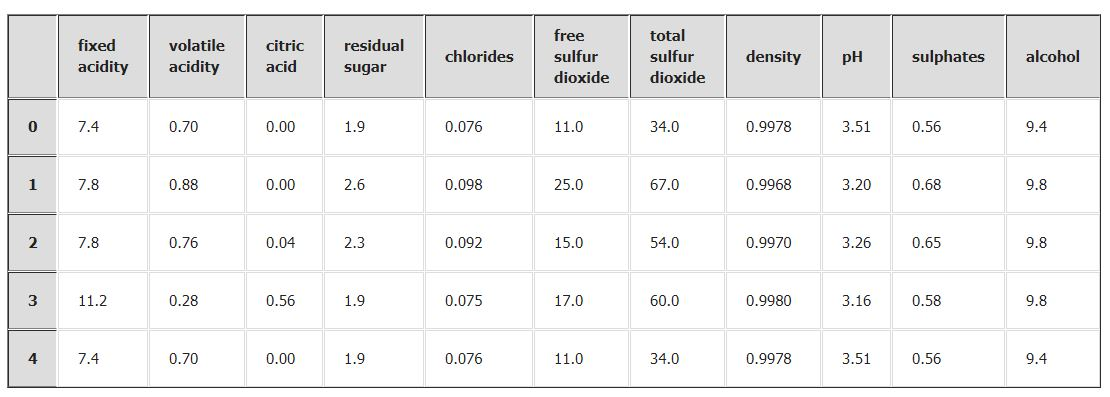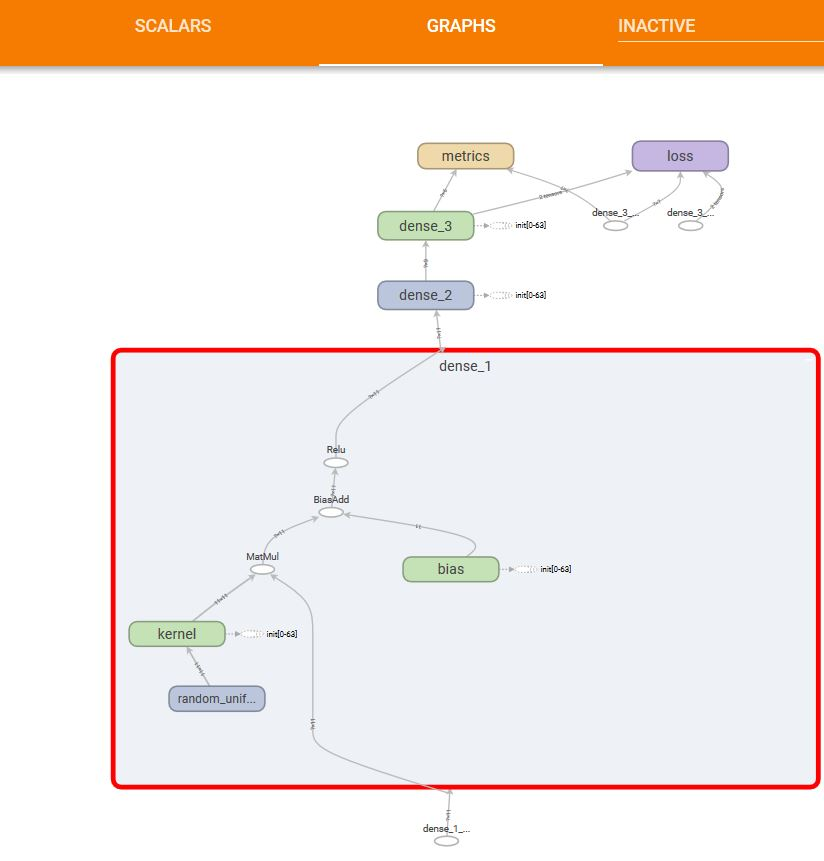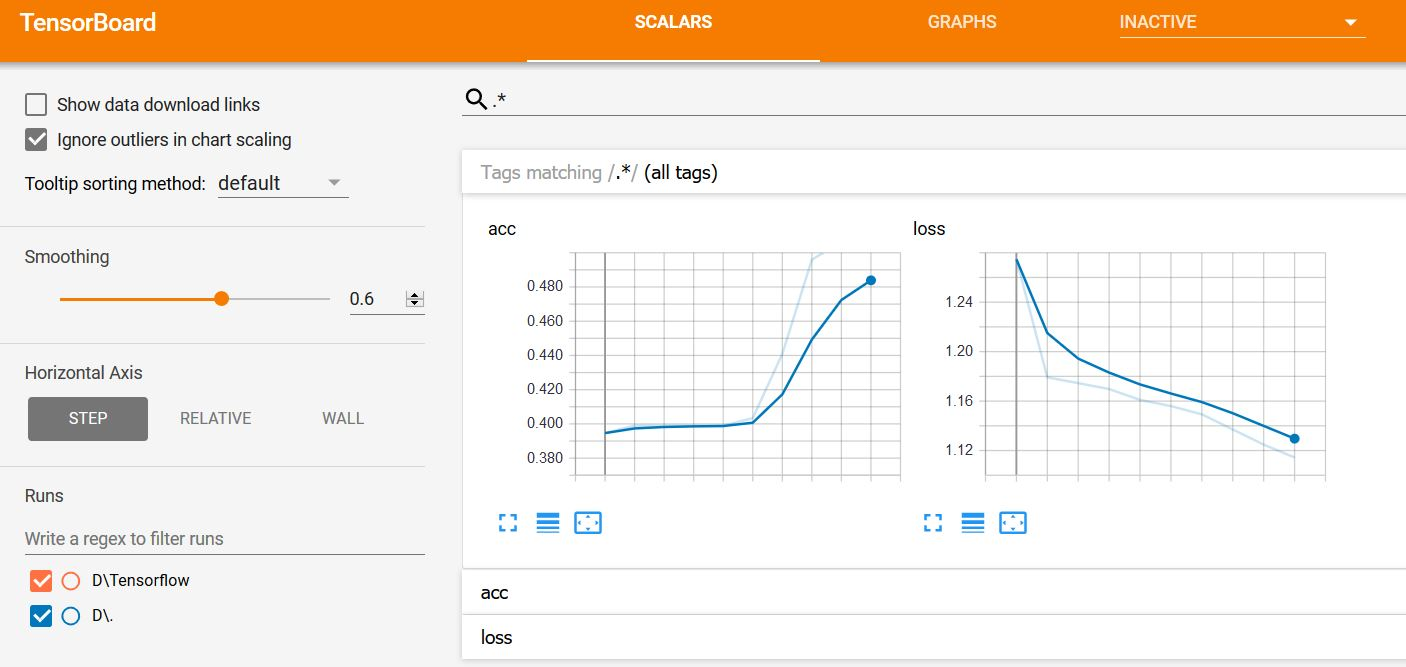# Classification

Classification is a technique in which we classify series of objects. Let us assume we have six categories of samples of wine and we need to make a model to classify the wine quality.

Import the libraries required for the analysis,

```import numpy as np
import pandas as pd
from keras.models import Sequential
from keras.layers import Dense,Dropout
from sklearn.model_selection import train_test_split
from sklearn.model_selection import cross_val_score
from sklearn.model_selection import KFold
from sklearn.preprocessing import LabelEncoder```

```df=pd.read_csv("winequality-red.csv")
X=df.iloc[:,0:11].values
y=df.iloc[:,-1].values
```Now , transform the data to be fit into a deep learning model,

```from keras.utils import np_utils
encoder = LabelEncoder()
encoder.fit(y)
encoded_Y = encoder.transform(y)
# convert integers to dummy variables
# convert integers to dummy variables (i.e. one hot encoded)
dummy_y = np_utils.to_categorical(encoded_Y)
dummy_y.shape```

Create a model, this model is having 11 input and 6 as the categorical shape as we have encoded it with categorical encoding( refer shape above)

```def baseline_model():
# create model
model = Sequential()
# Compile model
return model
```

Using K-Fold method,

```from keras.wrappers.scikit_learn import KerasClassifier
estimator = KerasClassifier(build_fn=baseline_model, epochs=100, batch_size=100, verbose=0)
kfold = KFold(n_splits=10, shuffle=True, random_state=seed)
results = cross_val_score(estimator, X, dummy_y, cv=kfold)
print("Accuracy: %.2f%% (%.2f%%)" % (results.mean()*100, results.std()*100))```
`Accuracy: 56.85% (2.52%)`

Using simple modeling technique,

```model = Sequential()
# Compile model
model.fit(X, dummy_y)```
```Epoch 1/10
1599/1599 [==============================] - 1s - loss: 1.3069 - acc: 0.4221
Epoch 2/10
1599/1599 [==============================] - 0s - loss: 1.1760 - acc: 0.4909
Epoch 3/10
1599/1599 [==============================] - 0s - loss: 1.1731 - acc: 0.4209
Epoch 4/10
1599/1599 [==============================] - 0s - loss: 1.1622 - acc: 0.4459
Epoch 5/10
1599/1599 [==============================] - 0s - loss: 1.1571 - acc: 0.4928
Epoch 6/10
1599/1599 [==============================] - 0s - loss: 1.1346 - acc: 0.5003
Epoch 7/10
1599/1599 [==============================] - 0s - loss: 1.1211 - acc: 0.5116
Epoch 8/10
1599/1599 [==============================] - 0s - loss: 1.1210 - acc: 0.5003
Epoch 9/10
1599/1599 [==============================] - 0s - loss: 1.1077 - acc: 0.5078
Epoch 10/10
1599/1599 [==============================] - 0s - loss: 1.0982 - acc: 0.5009

```

The Graph of the model is as:The loss is as,